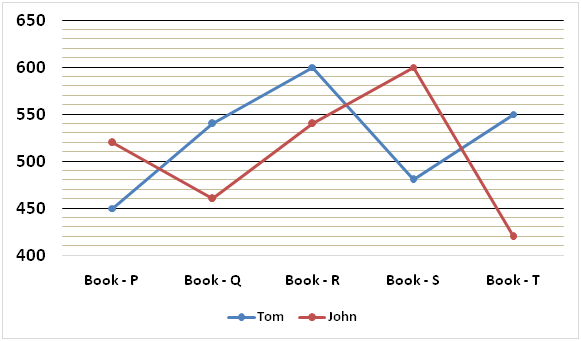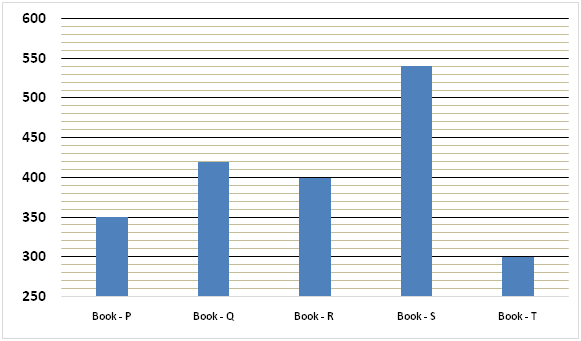# SBI PO Prelims Quantitative Aptitude Questions 2019 – Day 01

SBI PO 2019 Notification will be expected soon. It is one of the most expected recruitment among the banking aspirants. Every year the exam pattern for SBI PO has been changing. Depends upon the changing of exam pattern the questions are quite harder compare to the previous year. So the questions are in high level than the candidate’s assumption.

As per the latest trend, our IBPS Guide is providing the updated New Exam Pattern Quantitative Aptitude questions for SBI PO 2019 Day 1. Our Skilled experts were mounting the questions based on the aspirant’s needs. So candidates shall start your preparation and practice on daily basis with our SBI PO pattern quantitative aptitude questions 2019 day 1. Start your effective preparation from the right beginning to get success in upcoming SBI PO 2019.

[WpProQuiz 4962]

### Click Here for SBI PO Pre 2019 High-Quality Mocks Exactly on SBI Standard

Directions (1 – 5): Study the following information carefully and answer the questions given below:

The following line graph represents number of five different books sold by two shopkeepers in the month of March.The following bar graph represents cost prices of the books.1) Number of Book – P sold by Tom in April is 20% more than that of previous month and number of Book – P sold by John in April is 300 more than that of previous month. Find the total number of Book – P sold by Tom and John in April.

a) 1080

b) 1530

c) 1360

d) 1240

e) None of these

2) Tom sold each of Book – Q for Rs.450 and each of Book – R for Rs.420. Find the approximate overall percent profit earned by Tom by selling Book – Q and Book – R.

a) 10%

b) 8%

c) 6%

d) 9%

e) 12%

3) Find the respective ratio of total number of all the five books sold by Tom in March and total number of all the five books sold by John in March.

a) 139:133

b) 131:127

c) 129:121

d) 135:131

e) None of these

4) Tom sold each of Book – S at a profit of 10% on cost price and John sold each of Book – S at a profit of 8% on cost price. Find the difference between the selling price of all the Book – S for Tom and John.

a) Rs.38600

b) Rs.44500

c) Rs.64800

d) Rs.56600

e) None of these

5) Total cost of all the Book – T for John is approximately what percent of the total cost of all the Book – R for Tom?

a) 32%

b) 46%

c) 44%

d) 35%

e) 53%

6) Four friends Smriti, Geeta, Suman and Rupam jog around a circular field and complete one round in 10, 15, 16 and 20 seconds respectively. In what time all the four friends will meet again at the starting point?

a) 8 minutes

b) 4 minutes

c) 2 minutes

d) 6 minutes

e) None of these

7) Sushil and Manoj together can complete a piece of work in 60/7 days. Sushil alone can complete the work in 5 days less than the time taken by Manoj to complete the work alone. Lali alone can complete the work in 40 days. Find the number of days taken by Sushil and Lali to complete the work together.

a) 90/7 days

b) 120/11 days

c) 90/11 days

d) 120/7 days

e) None of these

8) Sumedha bought 500 toy cars for Rs.200 each and she also bought 800 toy bikes for Rs.400 each. She sold half of the toy cars at a profit of 20% and rest half at a loss of 10%. She sold 40% of the toy bikes at a loss of 20% and rest at a profit of 30%. Find her overall approximate profit percent.

a) 7%

b) 15%

c) 6%

d) 11%

e) 9%

9) Average of the present ages of Rahul and Nisha is 4 years more than the average of the present ages of Nisha and Saumya. Respective ratio of the ages of Nisha and Saumya before four years was 5:9. After two years, respective ratio of their ages will be 13:21. Find the present age of Rahul.

a) 40 years

b) 48 years

c) 52 years

d) 44 years

e) None of these

10) Two unbiased dice are thrown simultaneously. Find the product of the probability that sum of the numbers on both the dice are prime and probability that sum of the numbers on both the dice are composite.

a) 33/108

b) 33/133

c) 35/72

d) 35/144

e) None of these

Direction (1-5) :

Number of Book – P sold by Tom in March = 450

Number of Book – P sold by Tom in April = 450 x 120/100 = 540

Number of Book – P sold by John in March = 520

Number of Book – P sold by John in April = 520 + 300 = 820

Required sum = 540 + 820 = 1360

Total cost price of all the Book – Q and Book – R for Tom

= (540 x 420) + (600 x 400)

= 226800 + 240000

= Rs.466800

Total selling price of all the Book – Q and Book – R for Tom

= 540 x 450 + 600 x 420

= 243000 + 252000

= Rs.495000

% profit = (495000 – 466800)/466800 x 100

= 28200/466800 x 100

= 6.04%

= 6% approx.

Total number of all the five books sold by Tom in March

= 450 + 540 + 600 + 480 + 550 = 2620

Total number of all the five books sold by John in March

= 520 + 460 + 540 + 600 + 420 = 2540

Required ratio = 2620: 2540 = 131: 127

Cost price of Book – S = Rs.540

Selling price of all the Book – S for Tom = 480 x 540 x 110/100 = Rs.285120

Selling price of all the Book – S for John = 600 x 540 x 108/100 = Rs.349920

Required difference = 349920 – 285120 = Rs.64800

Total cost of all the Book – T for John = 420 x 300 = Rs.126000

Total cost of all the Book – R for Tom = 600 x 400 = Rs.240000

Required percentage = 126000/240000 x 100 = 52.5% = 53% approx.

Time after which they will meet again at the starting point

= LCM of 10, 15, 16 and 20

= 240 seconds

= 240/60 minutes

= 4 minutes

Let, number of days taken by Manoj to complete the work = n

=> Number of days taken by Sushil to complete the work = n – 5

1/n + 1/(n – 5) = 7/60

=> (n – 5 + n)/(n2 – 5n) = 7/60

=> (2n – 5)/(n2 – 5n) = 7/60

=> 120n – 300 = 7n2 – 35n

=>7n2 – 35n – 120n + 300 = 0

=> 7n2 – 155n + 300 = 0

=> 7n2 – 140n – 15n + 300 = 0

=>7n (n – 20) – 15(n – 20) = 0

=> (7n – 15)(n – 20) = 0

=> n = 20, 15/7 (rejected)

=> n = 20

Number of days taken by Sushil to complete the work = 20 – 5 = 15

Let, required number of days = k

k x (1/15 + 1/40) = 1

=> k x (8 + 3)/120 = 1

=> k = 120/11 days

Total cost price = (500 x 200) + (800 x 400)

= 100000 + 320000

= Rs.420000

Total selling price of toy cars

= (500/2 x 200 x 120/100) + (500/2 x 200 x 90/100)

= 60000 + 45000

= Rs.105000

Total selling price of toy bikes

= 40/100 x 800 x 400 x 80/100 + 60/100 x 800 x 400 x 130/100

= 102400 + 249600

= Rs.352000

Total selling price = 105000 + 352000 = Rs.457000

% profit = (457000 – 420000)/420000 x 100

= 37000/420000 x 100

= 8.809%

= 9% approx.

Let, ages of Nisha and Saumya before four years be 5k years and 9k years respectively.

(5k + 6)/(9k + 6) = 13/21

=> 105k + 126 = 117k + 78

=> 117k – 105k = 126 – 78

=> 12k = 48

=> k = 48/12

=> k = 4

Present age of Nisha = 5k + 4 = 5 x 4 + 4 = 24 years

Present age of Saumya = 9k + 4 = 9 x 4 + 4 = 40 years

Average of the present ages of Nisha and Saumya = (24 + 40)/2

= 64/2

= 32 years

Nisha + Rahul = (32 + 4) x 2

=>24 + Rahul = 72

=> Rahul = 72 – 24

=> Rahul = 48 years

Total number of outcomes = 6 x 6

Favourable outcomes for sum of the numbers on both the dice are prime

= {(1,1), (1,2), (1,4), (1,6), (2,1), (2,3), (2,5), (3,2), (3,4), (4,1), (4,3), (5,2), (5,6), (6,1), (6,5)}

No. of favourable outcomes = 15

Probability that sum of the numbers on both the dice are prime = 15/36 = 5/12

Favourable outcomes for sum of the numbers on both the dice are composite

= {(1,3), (1,5), (2,2), (2,4), (2,6), (3,1), (3,3), (3,5), (3,6), (4,2), (4,4), (4,5), (4,6), (5,1), (5,3), (5,4), (5,5), (6,2), (6,3), (6,4), (6,6)}

No. of favourable outcomes = 21

Sum of the numbers on both the dice are composite = 21/36 = 7/12

Required product = 5/12 x 7/12 = 35/144

### Click Here for SBI PO Pre 2019 High-Quality Mocks Exactly on SBI Standard